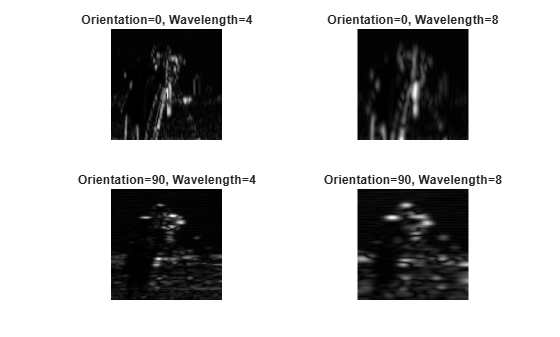Documentation

This is machine translation

Mouseover text to see original. Click the button below to return to the English version of the page.

imgaborfilt

Apply Gabor filter or set of filters to 2-D image

Syntax

``````[mag, phase] = imgaborfilt(A,wavelength,orientation)``````
``[mag, phase] = imgaborfilt(A,wavelength,orientation,Name,Value,...)``
``````[mag, phase] = imgaborfilt(A,gaborbank)``````

Description

example

``````[mag, phase] = imgaborfilt(A,wavelength,orientation)``` computes the magnitude and phase response of a Gabor filter for the input grayscale image `A`. `wavelength` describes the wavelength in pixels/cycle of the sinusoidal carrier. `orientation` is the orientation of the filter in degrees. The output `mag` and `phase` are the magnitude and phase responses of the Gabor filter.```
````[mag, phase] = imgaborfilt(A,wavelength,orientation,Name,Value,...)` applies a single Gabor filter using name-value pairs to control various aspects of filtering.```

example

``````[mag, phase] = imgaborfilt(A,gaborbank)``` applies the array of Gabor filters, `gaborbank`, to the input image `A`. `gaborbank` is a 1-by-p array of Gabor objects, called a filter bank. `mag` and `phase` are image stacks where each plane in the stack corresponds to one of the outputs of the filter bank. For inputs of size `A`, the outputs `mag` and `phase` contain the magnitude and phase response for each filter in `gaborbank` and are of size m-by-n-by-p. Each plane in the magnitude and phase responses, `mag(:,:,ind),phase(:,:,ind)`, is the result of applying the Gabor filter of the same index, `gaborBank(ind)`.```

Examples

collapse all

`I = imread('board.tif');`

Convert image to grayscale.

`I = rgb2gray(I);`

Apply Gabor filter to image.

```wavelength = 4; orientation = 90; [mag,phase] = imgaborfilt(I,wavelength,orientation);```

Display original image with plots of the magnitude and phase calculated by the Gabor filter.

```figure subplot(1,3,1); imshow(I); title('Original Image'); subplot(1,3,2); imshow(mag,[]) title('Gabor magnitude'); subplot(1,3,3); imshow(phase,[]); title('Gabor phase');````I = imread('cameraman.tif');`

Create array of Gabor filters, called a filter bank. This filter bank contains two orientations and two wavelengths.

`gaborArray = gabor([4 8],[0 90]);`

Apply filters to input image.

`gaborMag = imgaborfilt(I,gaborArray);`

Display results. The figure shows the magnitude response for each filter.

```figure subplot(2,2,1); for p = 1:4 subplot(2,2,p) imshow(gaborMag(:,:,p),[]); theta = gaborArray(p).Orientation; lambda = gaborArray(p).Wavelength; title(sprintf('Orientation=%d, Wavelength=%d',theta,lambda)); end```Input Arguments

collapse all

Input grayscale image, specified as a real, nonsparse 2-D matrix.

If the image contains `Inf`s or `NaN`s, the behavior of `imgaborfilt` is undefined because Gabor filtering is performed in the frequency domain.

For all input data types other than `single`, `imgaborfilt` performs the computation in `double`. Input images of type `single` are filtered in type `single`. Performance optimizations may result from casting the input image to `single` prior to calling `imgaborfilt`.

Data Types: `single` | `double` | `int8` | `int16` | `int32` | `int64` | `uint8` | `uint16` | `uint32` | `uint64`

Wavelength of the sinusoidal carrier, specified as a numeric scalar in the range `[2,Inf)`, in pixels/cycle.

Data Types: `single` | `double` | `int8` | `int16` | `int32` | `int64` | `uint8` | `uint16` | `uint32` | `uint64`

Orientation of filter in degrees, specified as a numeric scalar in the range `[0 360]`, where the orientation is defined as the normal direction to the sinusoidal plane wave.

Data Types: `single` | `double` | `int8` | `int16` | `int32` | `int64` | `uint8` | `uint16` | `uint32` | `uint64`

Array of Gabor filters, specified as a `gabor` object. You must use the `gabor` function to create an array of Gabor filters.

Name-Value Pair Arguments

Specify optional comma-separated pairs of `Name,Value` arguments. `Name` is the argument name and `Value` is the corresponding value. `Name` must appear inside quotes. You can specify several name and value pair arguments in any order as `Name1,Value1,...,NameN,ValueN`.

Example: `[mag,phase] = imgaborfilt(I,4,90,'SpatialFrequencyBandwidth',2);`

Spatial frequency bandwidth, specified as a numeric scalar in units of octaves. The spatial frequency bandwidth determines the cutoff of the filter response as frequency content in the input image varies from the preferred frequency, 1/lambda. Typical values for spatial-frequency bandwidth are in the range ```[0.5 2.5]```.

Data Types: `single` | `double` | `int8` | `int16` | `int32` | `int64` | `uint8` | `uint16` | `uint32` | `uint64`

Ratio of semi-major and semi-minor axes of Gaussian envelope (`semi-minor/semi-major`), specified as a numeric scalar. This parameter controls the ellipticity of the Gaussian envelope. Typical values for spatial aspect ratio are in the range ```[0.23 0.92]```.

Data Types: `single` | `double` | `int8` | `int16` | `int32` | `int64` | `uint8` | `uint16` | `uint32` | `uint64`

Output Arguments

collapse all

Magnitude response for the Gabor filter, returned as a numeric array of class `double`.

Phase response for the Gabor filter, returned as a numeric array of class `double`.